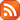Subscribe by RSS

# Operator Algebra Seminars

Fri., Nov. 3, 2017 10:00 a.m. - Fri., Nov. 3, 2017 11:00 p.m.

Location: RIC 215

Speaker: Xiao Xiong, University of Saskatchewan

Title: Similarity degree for twisted Fourier algebras

﻿Abstract:

Let $G$ by a locally compact group, and $\sigma$ a normalized 2-cocycle on $G$. Denote by $C^*(G,\sigma)$, $C_r^*(G,\sigma)$ and $VN(G,\sigma)$ the twisted full $C^*$-algebra, reduced algebra and von Neumann algebra respectively, and $B(G,\sigma)$ the dual of $C^*(G,\sigma)$ and $A(G,\sigma)$ the predual of $VN(G,\sigma)$. For nontrivial $\sigma$, $B(G,\sigma)$ is not a Banach algebra any more. But we still have $B(G,\sigma)\subset M_{cb}\big(A(G),A(G,\sigma)\big) \subset M\big(A(G),A(G,\sigma)\big)$, where $M$ (resp. $M_{cb}$) denotes the space of (resp. completely) bounded Fourier multipliers. Moreover, the equivalence of the three characterizes the amenability of $G$. Applying this assertion, we prove the twisted analogue of similarity degree conclusion for group algebras obtained by Pisier and Spronk.